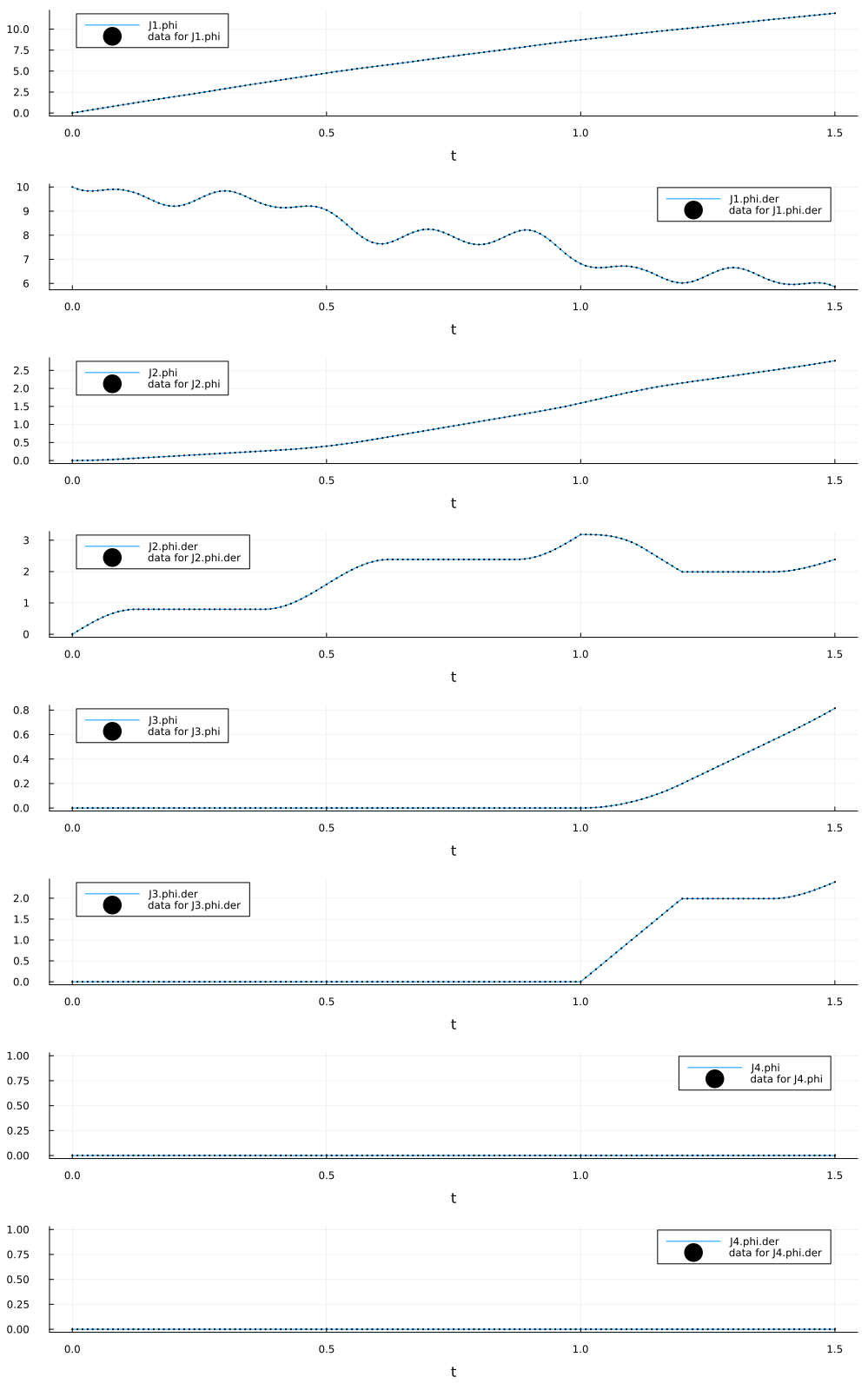# Calibrate a Functional Mock-up Unit (FMU)

In this example, we will calibrate a Functional Mock-up Unit (FMU), following Functional Mockup Interface v2.0, to experiment data using the SingleShooting method.

The example is based on a Coupled-Clutches model that is available as an FMU. This Coupled-Clutches FMU is a model of a clutch system with three clutches.

## Julia environment

Let us load the required packages.

using FMI
using DataFrames, CSV
using JuliaSimModelOptimizer
using Optimization, OptimizationNLopt
using Plots

We start off by loading the FMU using FMI.jl - an open-source julia package which enables working with FMUs. We specify the type of the FMU as FMI.fmi2TypeModelExchange since we intend to simualate the FMU in Model Exchange mode.

fmu = fmiLoad(joinpath(@__DIR__, "../assets/CoupledClutches_ME.fmu"); type = FMI.fmi2TypeModelExchange)
Model name:        CoupledClutches
Type:              0

We load the data from a CSV file using the DataFrames.jl package. The data is a time series of the states of the system, such as the angular velocity and acceleration of the inertias. The true values of the parameters are freqHz = 2.0 and T2 = 1.0.

data = CSV.read(joinpath(@__DIR__, "../assets/CoupledClutches_data.csv"), DataFrame)
freqHz_test = 2.0
T2_test = 1.0
5×9 DataFrame
RowtimestampJ1.phiJ1.phi.derJ2.phiJ2.phi.derJ3.phiJ3.phi.derJ4.phiJ4.phi.der
Float64Float64Float64Float64Float64Float64Float64Float64Float64
10.00.010.00.00.00.00.00.00.0
20.010.09955279.915840.0004993590.0997420.00.00.00.0
30.020.1984219.862870.001989630.1979210.00.00.00.0
40.030.2969059.838220.00444740.2929890.00.00.00.0
50.040.3952629.83650.007834020.3834460.00.00.00.0

## Experiment Setup

We next use the data to setup an experiment. We specify the time span of the Experiment and the relative tolerance of the solver. We also specify the InverseProblem of interest to us where we specify the parameters we want to recover and their bounds.

exp = Experiment(data, fmu; tspan = (0.0, 1.5), reltol = 1e-8)
invprob = InverseProblem(exp, fmu, ["freqHz" => (0.05, 3), "T2" => (0.2, 1.5)])
InverseProblem for Functional Mock-up Unit 2.0 with one experiment optimizing 2 parameters.


## Run Calibration

We run the calibration using the calibrate function. We specify the SingleShooting algorithm to use for the calibration. We also specify the adtype to be NoAD() as we will use a gradient free optimizer for the calibration process. We use GN_ISRES as the optimizer.

opt = OptimizationNLopt.NLopt.GN_ISRES()
alg = SingleShooting(maxiters = 30_000, optimizer = opt)
r = calibrate(invprob, alg, adtype = Optimization.SciMLBase.NoAD())
Calibration result computed in 3 minutes and 18 seconds. Final objective value: 0.0.
Optimization ended with MaxIters Return Code and returned.

┌────────┬─────┐
│ freqHz │  T2 │
├────────┼─────┤
│    2.0 │ 1.0 │
└────────┴─────┘


We can see the values of the calibration matches the true values of the parameters, i.e., freqHz = 2.0 and T2 = 1.0 from the data.

## Visualize Results

We visualize the results of the calibration by plotting the experiment data and the calibrated model.

plot(exp, invprob, r, show_data = true, legend = :best, ms = 0.2, layout = (8, 1), size = (1000, 1600), left_margin = 5Plots.mm)